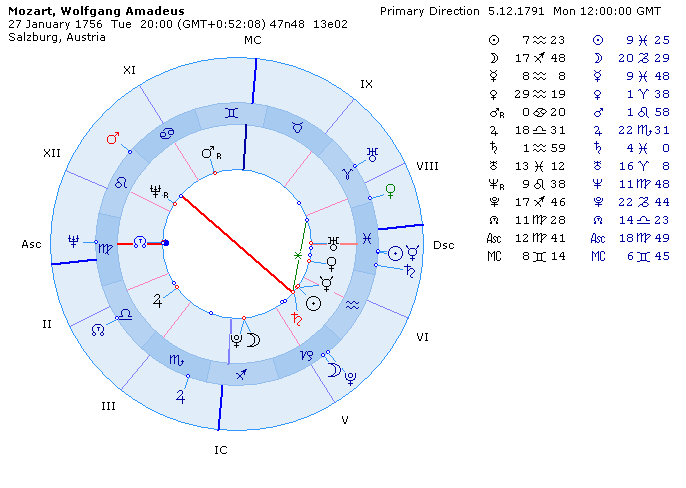Primary Directions Natural

 The principle of Primary Directions Natural is that one degree of movement of the celestial sphere relative to the horizon (Earth's axial rotation) corresponds to one revolution of the celestial sphere relative to the ecliptic (Earth's orbital rotation), i.e. 1 degree of right ascension (RA) of the MC = 1 tropical year, or one sidereal day = 360 tropical years. Primary Directions Natural are not an artifical 'symbolic' method, but a real projection of objects from one coordinate system to another. Let's look at the algorithm for calculating the Primary Directions Natural for Wolfgang Amadeus Mozart, born 27.01.1756 20:00 LMT, Salzburg, Austria in regard to the event of his death on 5.12.1791. Find the age (T) of the event: Td = JD - JDo [julian days], where JDo - natal Julian Date, JD - event date, Note: the Julian Date is an astronomical measure of time. JD = 2375548.00000 (date of event) JDo = 2362452.29713 (date of birth) Td = 13095.70287 julian days T = Td / 365.2422 [tropical years], Note: 1 tropical year = 365.2422 julian days, T = 35.85485 years.   Find the event's direction angle (Ad) in the proportion 1 degree of RA of MC = 1 tropical year: Ad = T * K * Kt [degrees], where K - the direction factor, defined with formula: number of sidereal days / number of tropical years * 365.2422, e.g., for Primary Direction 1 sidereal days = 360 years, K = 0.002770193, Kt - time direction factor: Kt = -1 means invers flow of time, Kt = +1 means direct flow of time. I.e. us = -35.75694° (-35°45'25").   Find the time (Ta) of real planet movement due to the Earth's axial rotation thru the event's angle Ad (astronomically this is a sidereal time), considering that 1 sidereal day = 360 degrees of right ascention: Ts = Ad / 360.98564736629 [tropical days], where 360.98564736629 is the factor for converting sidereal time to tropical time, Ts = -0.09905364 days (or 2h 22m 38s)   Calculate the right ascension (dRA) passed thru in this time span (Ta) by every planet due to it's own orbital speed, i.e. calculate the chart for the date JDo-Ta (progressed date). The minus sign indicates the fact that the orbital (zodiacal) movement is directed in reverse of the axial rotation. Notice that difference between natal (nRA) and progressed planet's positions (dRA) is quite small. Result of calculation (for Sun): nRA(Sun) = 309°47'43"             -0° 6' 9" dRA(Sun) = 309°41'34"   Find the planet and house cusp RA's by Primary Directions: RAi = dRAi + Ad; RA(Sun) = 309°41'34" + 35°45'25" = 345°26'59"   Convert the equatorial coordinates of planets to ecliptical ones using simple trigonometry formulae. The Primary Direction chart is ready. See below: